1067字，阅读需时4分钟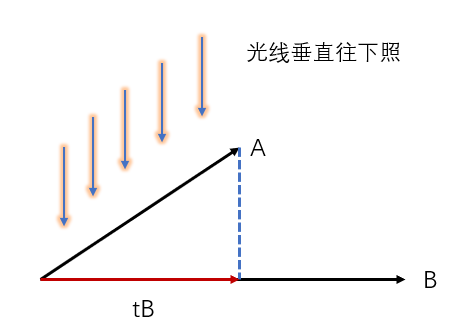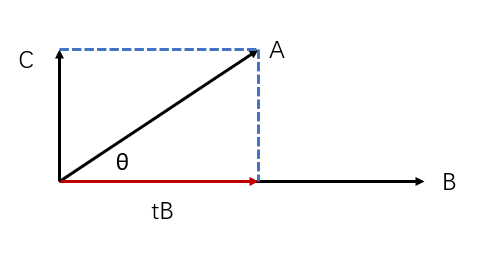tB + C = A

tB*B + C*B = A*B

tB*B = A * B

t为：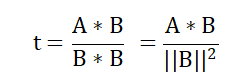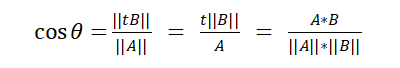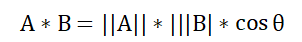```# 导入numpy模块
import  numpy as np

# 定义向量A
A = np.array([1,2,3])
# 定义向量B
B = np.array([1,2,2])

# 计算t
t = np.dot(A,B)/np.dot(B,B)
# 计算投影tB
tB = t * A
print(tB)```

```# 导入numpy模块
import  numpy as np

# 定义向量A和B
A = np.array([1,1,2,1,1,0,0])
B = np.array([0,1,2,1,0,1,1])

# 计算A和B的点积
AB = np.dot(A,B)
# 计算向量A的模长
mA = np.linalg.norm(A)
# 计算向量B的模长
mB = np.linalg.norm(B)
# 计算向量A和B的相似度
sim = AB/(mA*mB)
print('相似度为%.6f' % (sim))```